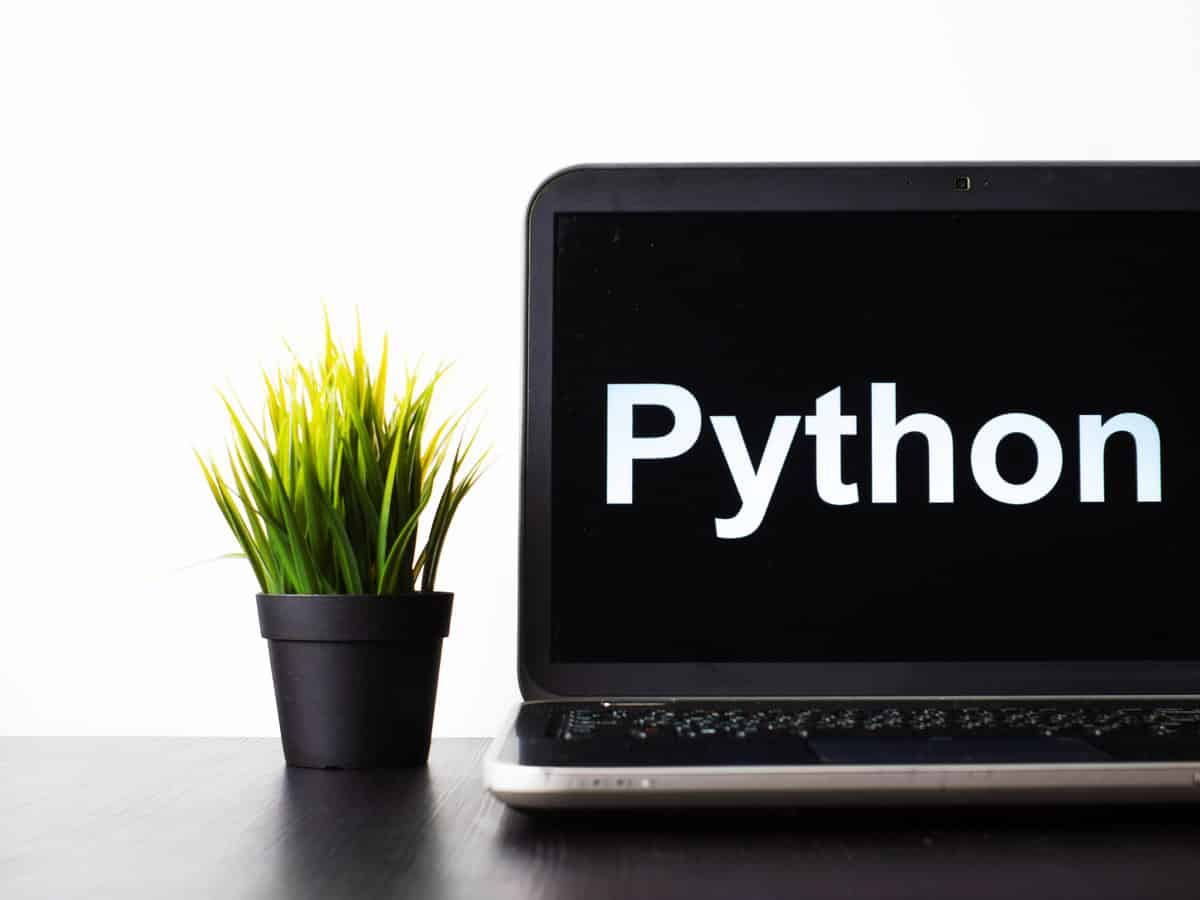# Python: Average Of List Of Numbers – Without Imports [One-Liner]How do you calculate the simple average of a list of numbers in Python, without needing to import any libraries?

Unfortunately, there’s no native ``` average ``` or ``` avg ``` function in Python to easily calculate the average of a list containing just numbers:

``````example_list = [1, 2, 3, 4, 5, 6]
average(example_list)
# NameError: name 'average' is not defined
avg(example_list)
# NameError: name 'avg' is not defined``````

To calculate the average of a list of numbers in Python, use the ``` sum ``` function and the ``` len ``` function and divide the total sum by the total length of the list to obtain the average, like so:

``````example_list = [1, 2, 3, 4, 5, 6]
simple_average = sum(example_list) / len(example_list)
print(simple_average)
# 3.5``````

## What if my list doesn’t contain any values?

Do be aware that if you have an empty list you will receive the following error:

``````empty_list = []
simple_average = sum(empty_list) / len(empty_list)
# ZeroDivisionError: division by zero``````

To catch instances where you’re passing through an empty list, you may want to perform a check on the length of the list, like so:

``````empty_list = []
simple_average = sum(empty_list) / len(empty_list) if len(empty_list) else None
print(simple_average)
# None``````

I’ve refrained from outputting a number, like ``` 0 ``` and instead have chosen ``` None ``` , but you could choose whatever alternative output you prefer.

## What if my list contains a ``` None ``` value or non-numeric value?

As the operation performed when calculating the average of a list of values assumes each value is a number, to get the average operation to work would require a filter to be placed on the list first , before passing through the filtered list for calculation.

The three types of numeric types in Python (currently) are: ``` int ``` , ``` float ``` and ``` complex ``` .

Therefore, we could loop through each element, check its value, and if it’s a number return a list of just the numbers:

``````dirty_list = [1, 2, 3, 4, 5, '6', None]
clean_list = list(filter(lambda x: type(x) in [int, float, complex], dirty_list))
simple_average = sum(clean_list) / len(clean_list) if len(clean_list) else None
print(clean_list)
# [1, 2, 3, 4, 5]
print(simple_average)
# 3``````

Notice with our first operation performed, we needed to clean the ``` dirty_list ``` first.

By using the ``` filter ``` method, combined with a ``` lambda ``` function we can set what we’re looking for to help calculate the average needed. The ``` lambda ``` function has a parameter defined as ``` x ``` (which ends up being each element in the original ``` dirty_list ``` and we perform a ``` type ``` check on that element to see if it is one of the accepted numeric values ``` int ``` , ``` float ``` , or ``` complex ``` .

The ``` filter ``` list method accepts a function on how to filter on each element for its first parameter, and then the list on what needs filtering as the second parameter.

As a ``` filter ``` method returns an object, we wrap this in a ``` list ``` method to translate the output from the ``` filter ``` method to a list.

Then we apply our standard formula for calculating the average of our new ``` clean_list ``` .

## Summary

To calculate the average of a list of numbers in Python, without importing any libraries, we use the ``` sum ``` function and then ``` len ``` function, and divide the ``` sum ``` total by the total number of elements (value returned from ``` len ``` ) in our list.

We do have to be careful to check for lists that may not contain any values, and it would be wise to place a condition to check the length of the list contains values.

Finally, we performed one other check on our list to make sure it contained numeric values to be able to calculate our simple average.

Author of scripteverything.com, Ryan has been dabbling in code since the late '90s when he cut his teeth by exploring VBA in Excel when trying to do something more. Having his eyes opened with the potential of automating repetitive tasks, he expanded to Python and then moved over to scripting languages such as HTML, CSS, Javascript and PHP. When he is not behind a screen, Ryan enjoys a good bush walk with the family during the cooler months, and going with them to the beach during the warmer months.